# Grade 3 Math STAAR Review Activities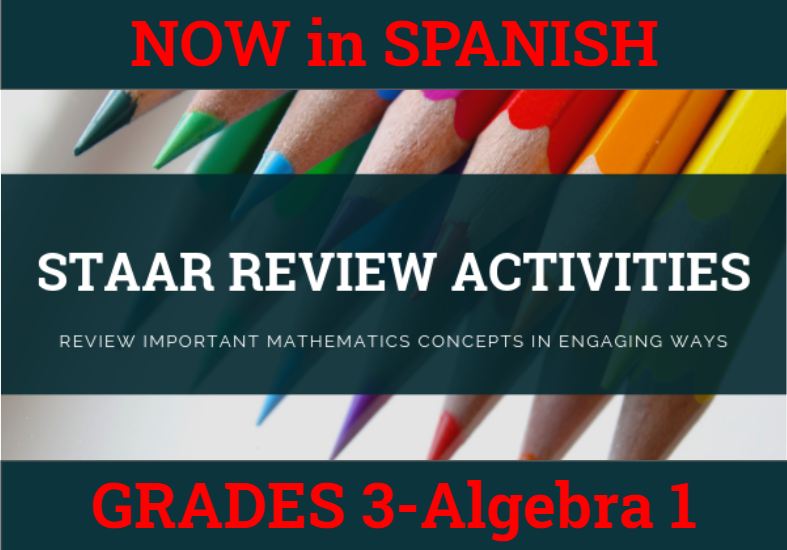How might we purposefully and effectively review important mathematics concepts for the Grade 3 Math STAAR assessment? The ESC-18 Math Team has created a variety of activities where students practice and apply important grade-level TEKS aligned topics to cement their learning.

 3.2A Composing & Decomposing Numbers up to 100,000 Question Stack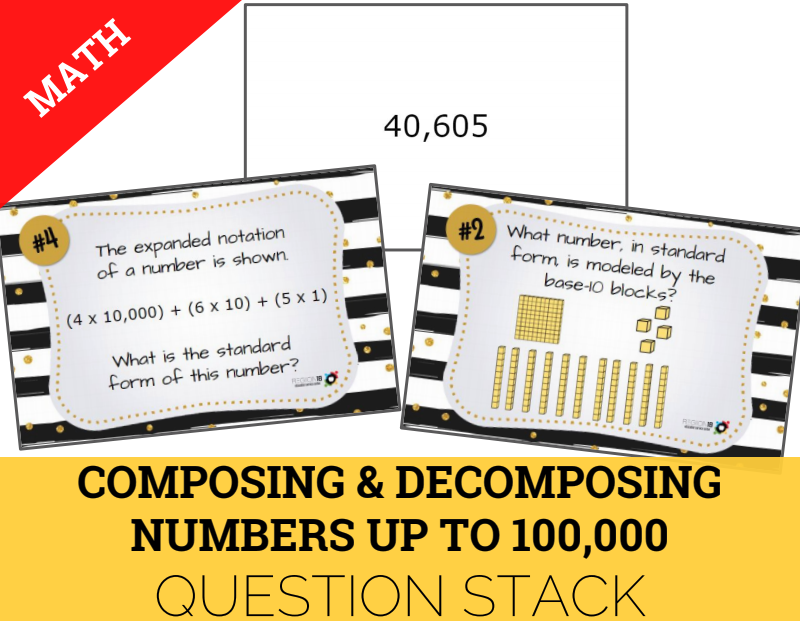Compose and decompose numbers up to 100,000 as a sum of so many ten thousands, thousands, hundreds, tens, and ones using objects, pictorial models, and numbers, including expanded notation as appropriate. 3.2D Comparing Whole Numbers True/False Sort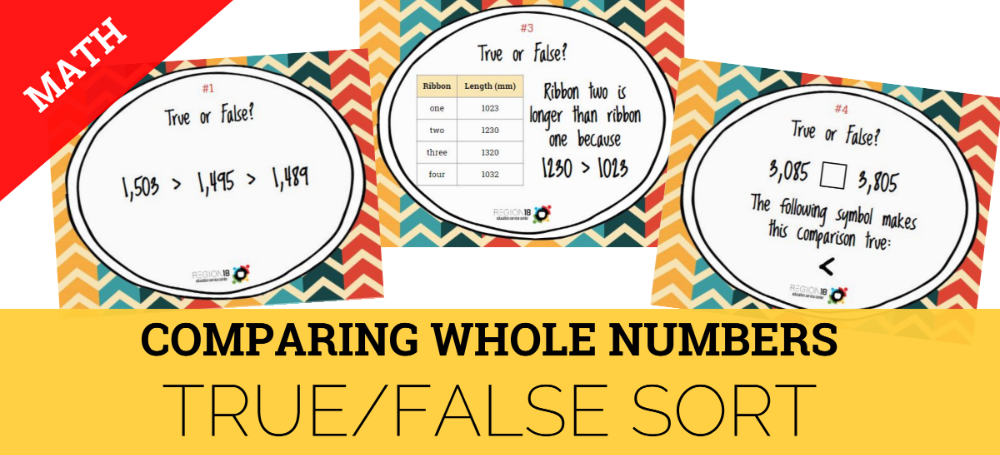Compare and order whole numbers up to 100,000 and represent comparisons using the symbols <, >, or =. 3.3F Representing Equivalent Fractions Dominoes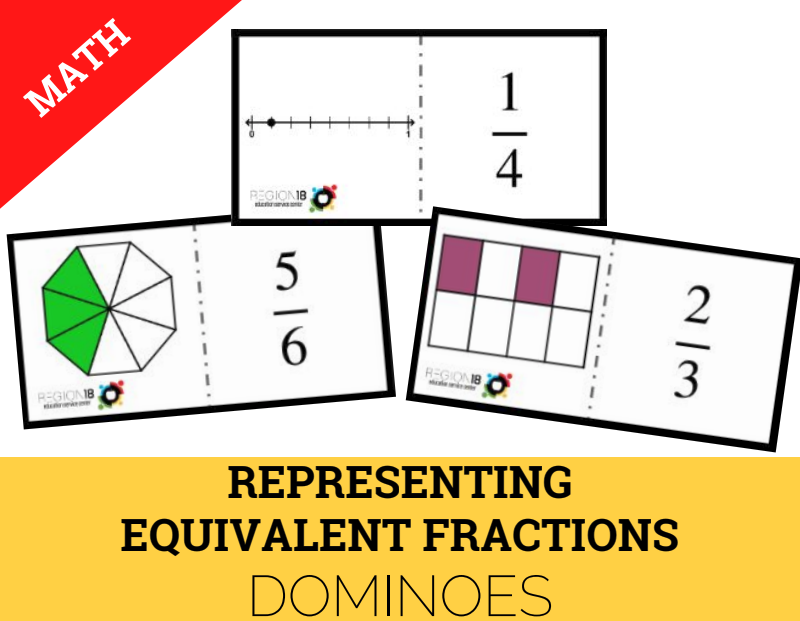Represent equivalent fractions with denominators 2, 3, 4, 6, and 8 using a variety of objects and pictorial models, including number lines. 3.3H Comparing Fractions Quick Flips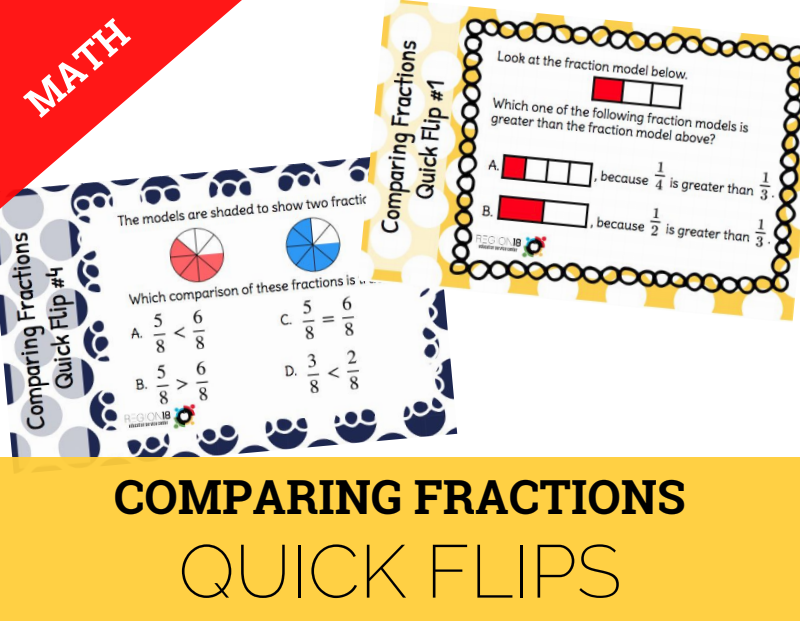Compare two fractions having the same numerator or denominator in problems by reasoning about their sizes and justifying the conclusion using symbols, words, objects, and pictorial models. 3.4A Addition & Subtraction Task Cards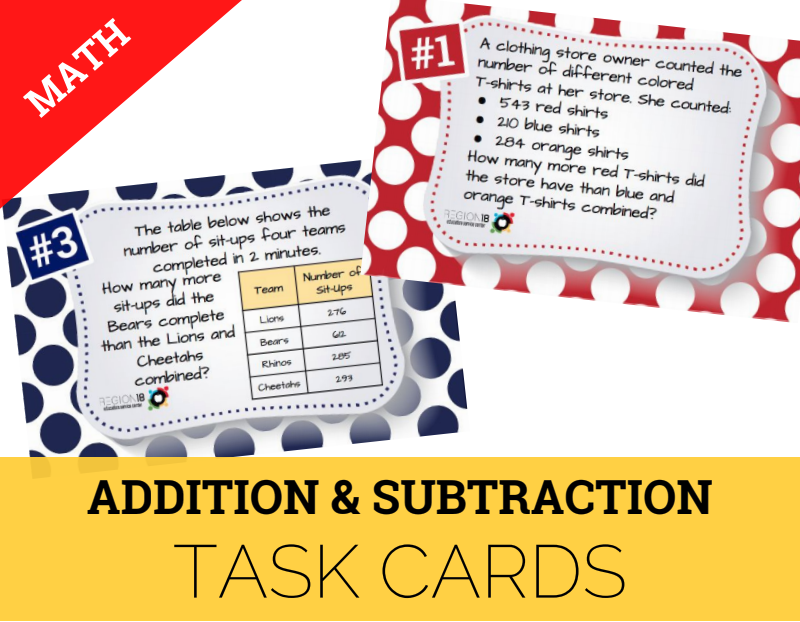Solve with fluency one-step and two-step problems involving addition and subtraction within 1,000 using strategies based on place value, properties of operations, and the relationship between addition and subtraction. 3.4K Multiplication & Division Scavenger Hunt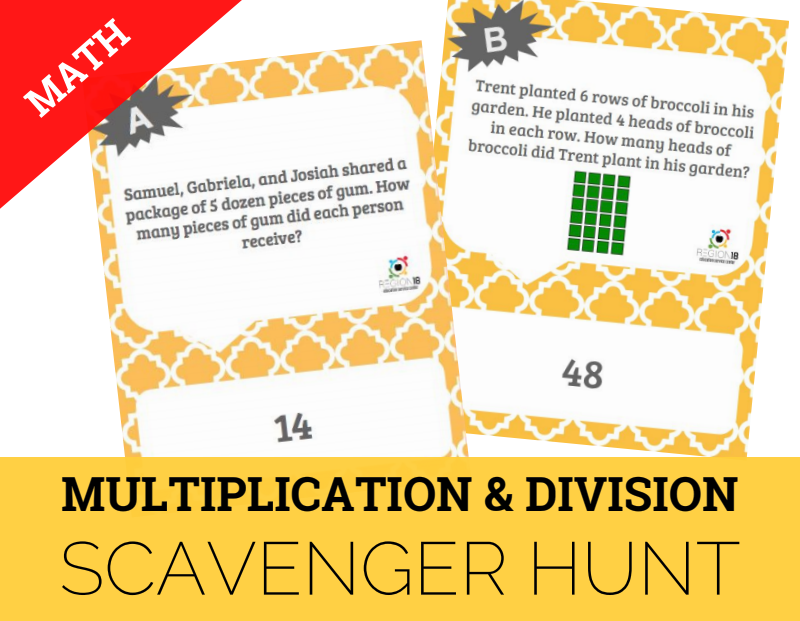Solve one-step and two-step problems involving multiplication and division within 100 using strategies based on objects; pictorial models, including arrays, area models, and equal groups; properties of operations; or recall of facts. 3.5A, 3.5B Representing Operations with Strip Diagrams Task Cards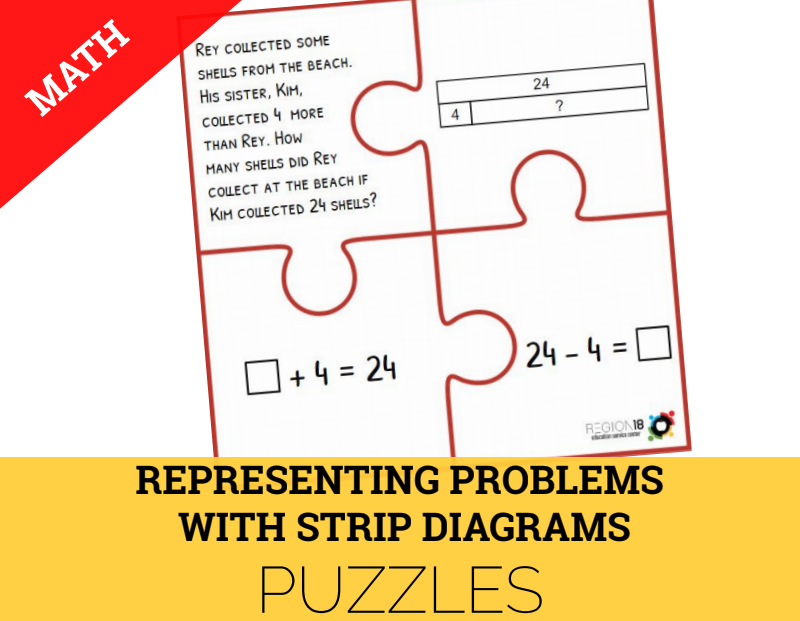Represent multi-step problems involving the four operations with whole numbers using strip diagrams and equations with a letter standing for the unknown quantity. 3.6C, 3.7B Perimeter & Area Sum It Up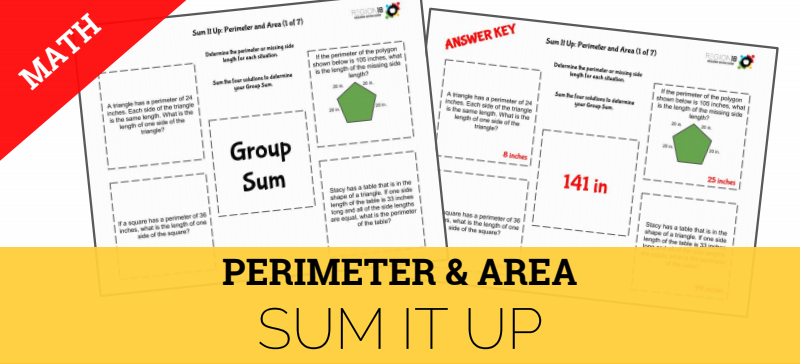Determine the area of rectangles with whole number side lengths in problems using multiplication related to the number of rows times the number of unit squares in each row. Determine the perimeter of a polygon or a missing length when given perimeter and remaining side lengths in problems. 3.6A 2D & 3D Shapes Spinner Bingo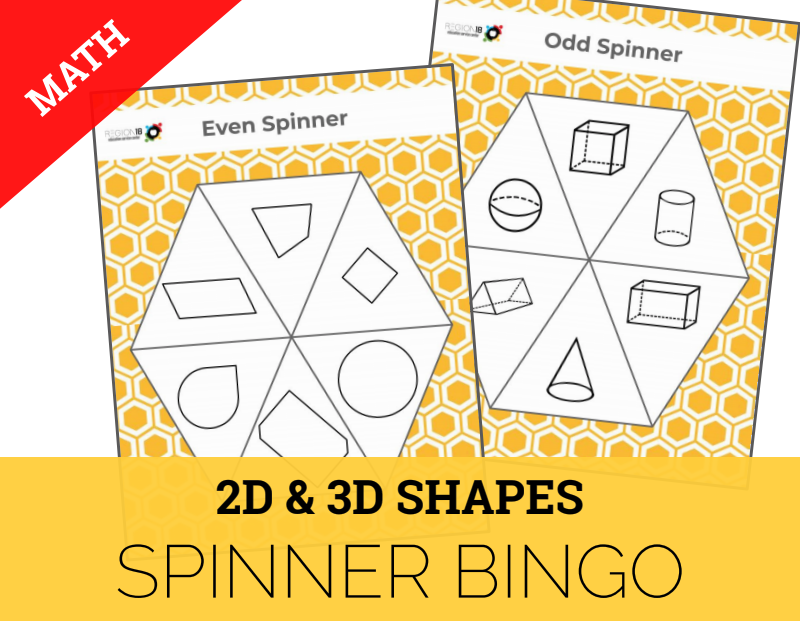Classify and sort two- and three-dimensional figures, including cones, cylinders, spheres, triangular and rectangular prisms, and cubes, based on attributes using formal geometric language. Mixed Review Grade 3 Griddable Practice Task Cards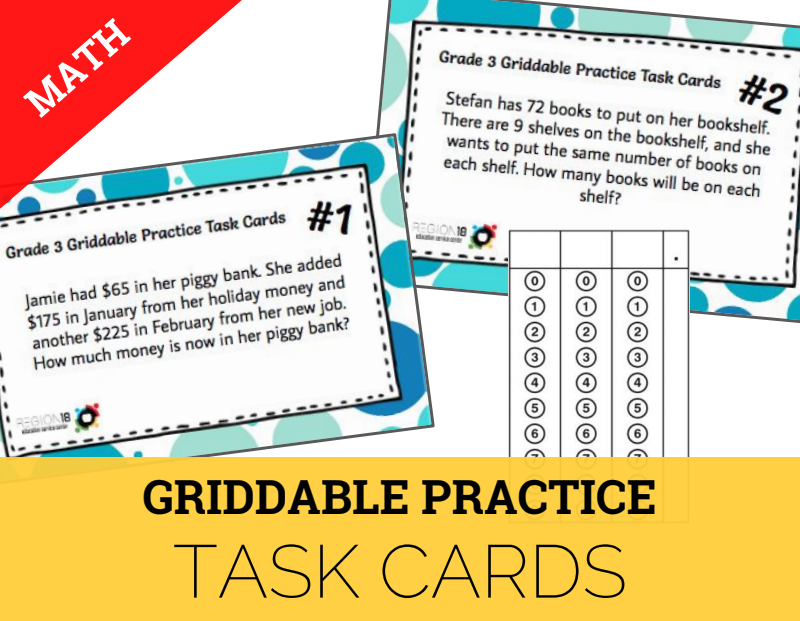Solve problems and record answers in the STAAR griddable format.